# deepSignalAnomalyDetector

Create signal anomaly detector

Since R2023a

## Syntax

``d = deepSignalAnomalyDetector(numChannels)``
``d = deepSignalAnomalyDetector(numChannels,modelType)``
``d = deepSignalAnomalyDetector(___,Name=Value)``

## Description

example

````d = deepSignalAnomalyDetector(numChannels)` creates a signal anomaly detector object `d` based on a 1-D convolutional autoencoder.```
````d = deepSignalAnomalyDetector(numChannels,modelType)` specifies the type of deep learning model implemented by the detector.```
````d = deepSignalAnomalyDetector(___,Name=Value)` specifies additional options using name-value arguments.```

## Examples

collapse all

Load the file `sineWaveAnomalyData.mat`, which contains two sets of synthetic three-channel sinusoidal signals.

`sineWaveNormal` contains 10 sinusoids of stable frequency and amplitude. Each signal has a series of small-amplitude impact-like imperfections. The signals have different lengths and initial phases. Plot the first three normal signals.

```load sineWaveAnomalyData.mat sl = 3; tiledlayout("vertical") ax = zeros(sl,1); for kj = 1:sl ax(kj) = nexttile; plot(sineWaveNormal{kj}) title("Normal Signal") end```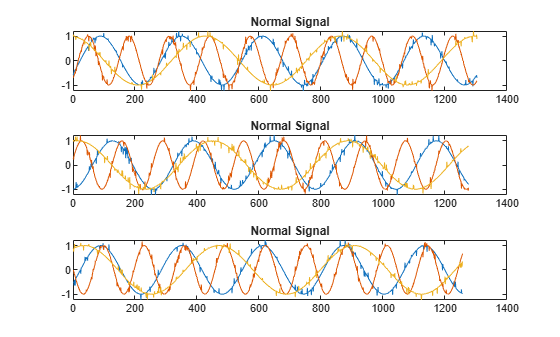`sineWaveAbnormal` contains three signals, all of the same length. Each signal in the set has one or more anomalies.

• All channels of the first signal have an abrupt change in frequency that lasts for a finite time.

• The second signal has a finite-duration amplitude change in one of its channels.

• The third signal has spikes at random times in all channels.

Plot the three signals with anomalies.

```for kj = 1:sl plot(ax(kj),sineWaveAbnormal{kj}) title(ax(kj),"Signal with Anomalies") end```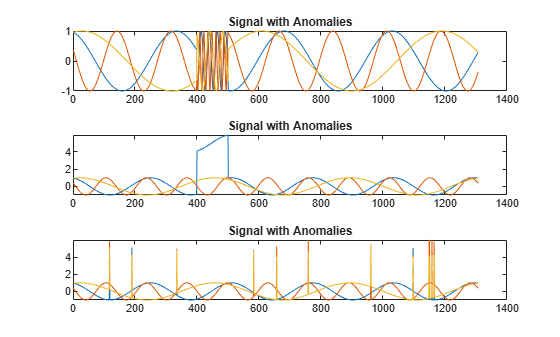Create an autoencoder object to detect the anomalies in the abnormal signals. The object contains a neural network that you can train to best reproduce an input set of anomaly-free data. The trained object encodes the patterns inherent in the data and can recognize if a signal contains regions that deviate from the patterns. You do not need to label any data to train the detector. The training process is unsupervised.

By default, the `deepSignalAnomalyDetector` function creates objects with convolutional autoencoders. The only mandatory argument is the number of channels in the training and testing signals.

`D = deepSignalAnomalyDetector(sl)`
```D = deepSignalAnomalyDetectorCNN with properties: IsTrained: 0 NumChannels: 3 Model Information ModelType: 'conv' FilterSize: 8 NumFilters: 32 NumDownsampleLayers: 2 DownsampleFactor: 2 DropoutProbability: 0.2000 Threshold Information Threshold: [] ThresholdMethod: 'contaminationFraction' ThresholdParameter: 0.0100 Window Information WindowLength: 1 OverlapLength: 'auto' WindowLossAggregation: 'mean' ```

Use the `trainDetector` function to train the convolutional neural network with the normal data. Use the training options for the adaptive moment estimation (Adam) optimizer. Specify a maximum number of 100 epochs to use for training. For more information, see `trainingOptions` (Deep Learning Toolbox).

```opts = trainingOptions("adam",MaxEpochs=100); trainDetector(D,sineWaveNormal,opts)```
```Training on single CPU. |========================================================================================| | Epoch | Iteration | Time Elapsed | Mini-batch | Mini-batch | Base Learning | | | | (hh:mm:ss) | RMSE | Loss | Rate | |========================================================================================| | 1 | 1 | 00:00:00 | 1.23 | 0.8 | 0.0010 | | 50 | 50 | 00:00:04 | 0.45 | 1.0e-01 | 0.0010 | | 100 | 100 | 00:00:08 | 0.38 | 7.2e-02 | 0.0010 | |========================================================================================| Training finished: Max epochs completed. Computing threshold... Threshold computation completed. ```

Use the trained detector to detect the anomalies in the abnormal data. The `detect` function outputs two cell arrays, with each array element corresponding to a signal in the testing data.

`[lbls,loss] = detect(D,sineWaveAbnormal);`

The first output of `detect` is a categorical array that declares each sample of a signal as being anomalous or not. A sample can be either a point, a signal region, or an entire signal. An anomaly is detected when the reconstruction loss, or the difference between the value of a signal and the value reconstructed by the detector based on the training data, exceeds a given threshold. You can set a threshold manually or you can direct the detector to compute a threshold based on a specified statistic of loss values computed on training data.

```for kj = 1:sl hold(ax(kj),"on") plot(ax(kj),lbls{kj},LineWidth=2) title(ax(kj),"Signal with Anomalies") hold(ax(kj),"off") end```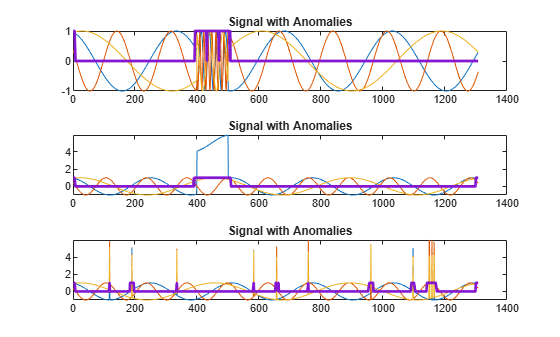Alternatively, use the `plotAnomalies` function to plot each channel of each signal and the anomalies found by the detector. The detector decides that there is an anomaly in a signal if it detects one in any of the signal channels. `plotAnomalies` plots the anomaly locations in all channels.

```for kj = 1:sl figure plotAnomalies(D,sineWaveAbnormal{kj}) end```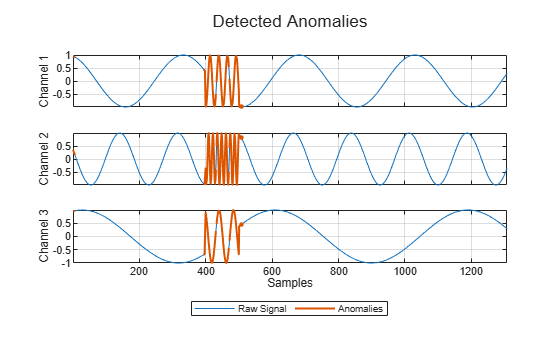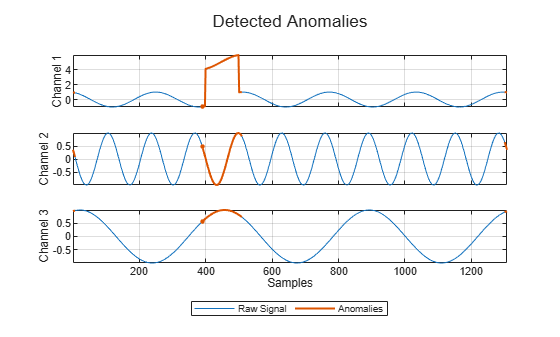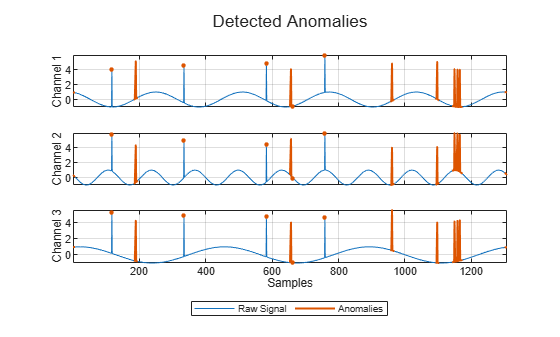The second output of `detect` is the computed reconstruction loss that the object uses to determine the labeling. You can plot the reconstruction loss directly from the output of `detect`, but it is more convenient to use the `plotLoss` function, which also displays the the threshold value above which a sample is declared to be anomalous.

```tiledlayout("vertical") for kj = 1:sl nexttile plotLoss(D,sineWaveAbnormal{kj}) end```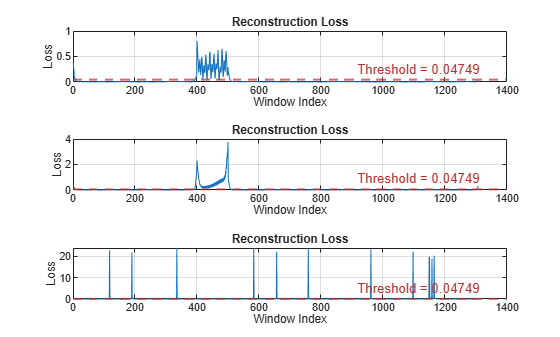The `plotLossDistribution` function displays the reconstruction loss distribution and gives insight into the way the detector chooses the threshold. You can plot the distribution loss for the normal data alongside the distribution loss for the abnormal data. The threshold chosen by the detector is larger than the loss values for most of the normal-data samples but smaller than an appreciable number of abnormal-data losses that signal the possible presence of anomalies. You can improve the choice of threshold by using the `updateDetector` function.

```clf plotLossDistribution(D,sineWaveNormal,sineWaveAbnormal)```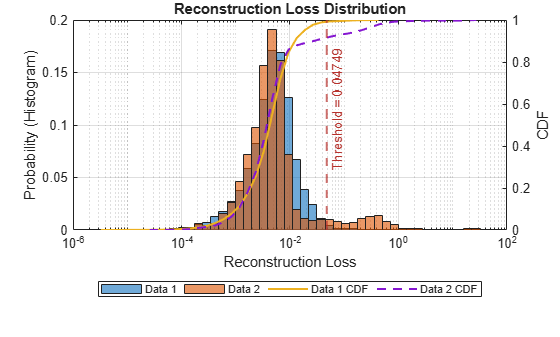Use the `getModel` function to obtain the underlying neural network model corresponding to the detector. You can then inspect the model using `analyzeNetwork` (Deep Learning Toolbox).

```M = getModel(D); analyzeNetwork(M)```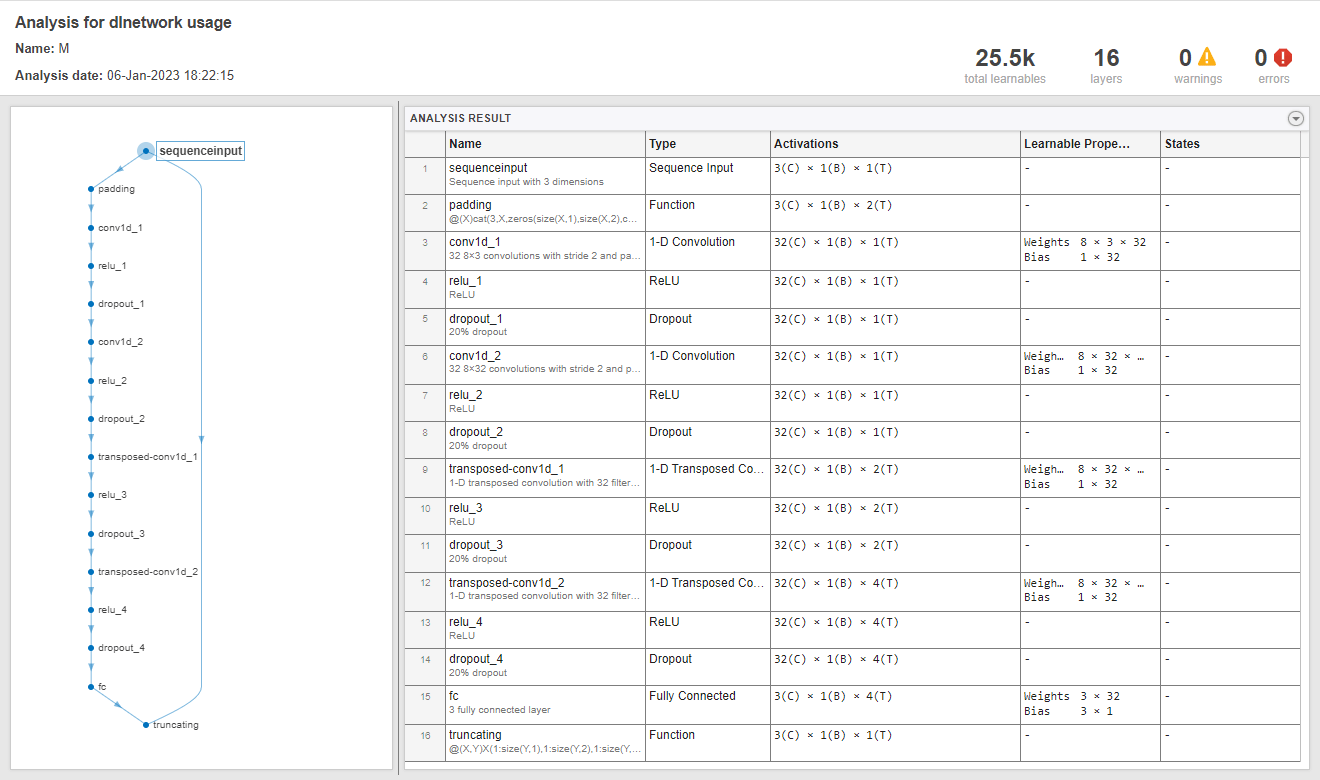## Input Arguments

collapse all

Number of channels in each signal input to the detector `d`, specified as a positive integer.

Data Types: `single` | `double` | `int8` | `int16` | `int32` | `int64` | `uint8` | `uint16` | `uint32` | `uint64`

Type of deep learning model implemented by the detector `d`, specified as `"conv"` or `"lstm"`. If you specify `"conv"`, the detector implements a 1-D convolutional autoencoder. If you specify `"lstm"`, the detector implements a long short-term memory (LSTM) autoencoder.

### Name-Value Arguments

Specify optional pairs of arguments as `Name1=Value1,...,NameN=ValueN`, where `Name` is the argument name and `Value` is the corresponding value. Name-value arguments must appear after other arguments, but the order of the pairs does not matter.

Example: `ThresholdMethod="manual",Threshold=0.4` specifies that the threshold value above which a sample is declared to be anomalous is set manually to `0.4`.

Window

collapse all

Window length of each signal segment, specified as a positive integer or as `"fullSignal"`.

• If you specify `WindowLength` as an integer, the detector divides each input signal into segments. The length of each segment is equal to the specified value in samples.

• If you specify `WindowLength` as `"fullSignal"`, the detector treats each input signal as a single segment.

Data Types: `single` | `double` | `int8` | `int16` | `int32` | `int64` | `uint8` | `uint16` | `uint32` | `uint64` | `char` | `string`

Number of overlapped samples between window segments, specified as a positive integer or as `"auto"`.

• If you specify `WindowLength` and `OverlapLength` as integers, the detector sets the number of overlapped samples to the specified value. The number of overlapped samples must be less than the window length.

• If you specify `WindowLength` as an integer and `OverlapLength` as `"auto"`, the detector sets the number of overlapped samples to `WindowLength` – 1.

• If you specify `WindowLength` as `"fullSignal"`, you cannot specify `OverlapLength` as an integer.

Data Types: `single` | `double` | `int8` | `int16` | `int32` | `int64` | `uint8` | `uint16` | `uint32` | `uint64` | `logical` | `char` | `string`

Method to aggregate sample loss within each window segment, specified as one of these:

• `"max"` — Compute the aggregated window loss as the maximum value of all the sample losses within the window.

• `"mean"` — Compute the aggregated window loss as the mean value of all the sample losses within the window.

• `"median"` — Compute the aggregated window loss as the median value of all the sample losses within the window.

• `"min"` — Compute the aggregated window loss as the minimum value of all the sample losses within the window.

The detector computes the detection loss of each sample within a window segment and aggregates the loss values over each window.

Threshold

collapse all

Method to compute the detection threshold, specified as one of these:

• `"contaminationFraction"` — Value corresponding to the detection of anomalies within a specified fraction of windows. The fraction value is specified by `ThresholdParameter`.

• `"max"` — Maximum window loss measured over the entire training data set and multiplied by `ThresholdParameter`.

• `"median"` — Median window loss measured over the entire training data set and multiplied by `ThresholdParameter`.

• `"mean"` — Mean window loss measured over the entire training data set and multiplied by `ThresholdParameter`.

• `"manual"` — Manual detection threshold value based on `Threshold`.

• `"customFunction"` — Custom detection threshold value based on `ThresholdFunction`.

If you specify `ThresholdMethod`, you can also specify `ThresholdParameter`, `Threshold`, or `ThresholdFunction`. The available threshold parameter depends on the specified detection method.

Detection threshold, specified as a real scalar.

• If `ThresholdMethod` is specified as `"max"`, `"mean"`, or `"median"`, specify `ThresholdParameter` as a positive scalar. If you do not specify `ThresholdParameter`, the detector sets the threshold to 1.

• If `ThresholdMethod` is specified as `"contaminationFraction"`, specify `ThresholdParameter` as a nonnegative scalar less than 0.5. If you do not specify `ThresholdParameter`, the detector sets the threshold to 0.01.

• If `ThresholdMethod` is specified as `"customFunction"` or `"manual"`, this argument does not apply.

Manual detection threshold, specified as a positive scalar. This argument applies only when `ThresholdMethod` is specified as `"manual"`.

Use this option when you do not want the detector to compute a threshold based on training data.

Data Types: `single` | `double` | `int8` | `int16` | `int32` | `int64` | `uint8` | `uint16` | `uint32` | `uint64`

Function to compute custom detection threshold, specified as a function handle. This argument applies only when `ThresholdMethod` is specified as `"customFunction"`.

• The function must have two inputs:

• The first input is a cell array of aggregated window loss values.

• The second input is a cell array of sample loss values before aggregation.

Each cell contains a loss vector for one signal observation.

• The function must return a positive scalar corresponding to the detection threshold.

Use this option when you want to compute a threshold based on training data.

Data Types: `function_handle`

Convolutional Model

collapse all

Number of convolutional layers in the downsampling section of the model, specified as a positive integer.

The model uses the same number of transposed convolutional layers in the upsampling section, with the parameters in reverse order.

Data Types: `single` | `double` | `int8` | `int16` | `int32` | `int64` | `uint8` | `uint16` | `uint32` | `uint64`

Downsampling factor used by convolutional layers, specified as a positive integer or a vector of positive integers.

• If you specify `DownsampleFactor` as a scalar, each layer uses the same downsampling factor.

• If you specify `DownsampleFactor` as a vector, the ith layer uses a downsampling factor equal to the value of the ith vector element. The length of the vector must be equal to the number of layers specified by `NumDownsampleLayers`.

Data Types: `single` | `double` | `int8` | `int16` | `int32` | `int64` | `uint8` | `uint16` | `uint32` | `uint64`

Number of filters in convolutional layers, specified as a positive scalar integer or a vector of positive integers.

• If you specify `NumFilters` as a scalar, each layer has the same number of filters.

• If you specify `NumFilters` as a vector, the ith layer has a number of filters equal to the value of the ith vector element. The length of the vector must be equal to the number of layers specified by `NumDownsampleLayers`.

Data Types: `single` | `double` | `int8` | `int16` | `int32` | `int64` | `uint8` | `uint16` | `uint32` | `uint64`

Size of filters in convolutional layers, specified as a positive integer or a vector of positive integers.

• If you specify `FilterSize` as a scalar, the size of each filter is the same in all layers.

• If you specify `FilterSize` as a vector, the size of the filters in the ith layer is equal to the value of the ith vector element. The length of the vector must be equal to the number of layers specified by `NumDownsampleLayers`.

Data Types: `single` | `double` | `int8` | `int16` | `int32` | `int64` | `uint8` | `uint16` | `uint32` | `uint64`

Dropout probability used to avoid overfitting, specified as a nonnegative scalar less than 1.

Data Types: `single` | `double` | `int8` | `int16` | `int32` | `int64` | `uint8` | `uint16` | `uint32` | `uint64`

LSTM Model

collapse all

Number of hidden units for each LSTM layer in the encoder, specified as a vector of positive integers. The ith element of the vector sets the number of hidden units in the ith layer.

Data Types: `single` | `double` | `int8` | `int16` | `int32` | `int64` | `uint8` | `uint16` | `uint32` | `uint64`

Number of hidden units in each LSTM layer of the decoder, specified as a vector of positive integers. The ith element of the vector sets the number of hidden units in the ith layer.

Data Types: `single` | `double` | `int8` | `int16` | `int32` | `int64` | `uint8` | `uint16` | `uint32` | `uint64`

## Output Arguments

collapse all

Signal anomaly detector, returned as a `deepSignalAnomalyDetectorCNN` object or a `deepSignalAnomalyDetectorLSTM` object. You must train `d` with data before you can use it to detect anomalies.

## Algorithms

collapse all

### Windows

To detect anomalies, `deepSignalAnomalyDetector` divides each channel of each signal into possibly overlapping windows. You can specify the window length using `WindowLength` and the number of overlap samples using `OverlapLength`.

• By default, the detector uses signals with a length of one sample and zero samples of overlap between adjoining windows. These settings detect point anomalies.

• You can also set `WindowLength` to `"fullSignal"` to detect whole-signal anomalies.

### Aggregated Loss

For each window, `deepSignalAnomalyDetector` computes sample-by-sample losses, defined as the square of the difference in value between the original signal and the reconstructed signal. The detector then aggregates the losses using the method specified using `WindowLossAggregation`. You can use the maximum sample loss, the minimum sample loss, the mean of the losses, or the median of the losses.

If the aggregated loss for a window is larger than a specified threshold, the object detects the whole window as abnormal.

### Threshold

To determine the threshold, `deepSignalAnomalyDetector` finds the aggregated losses for all the windows in the training data and combines them into a statistic. You can specify the method that the detector uses to compute the threshold using `ThresholdMethod`.

• The statistic can be the maximum value of the losses across the data, the minimum value, the mean value, or the median value. The threshold is the statistic multiplied by `ThresholdParameter`.

• If you expect a given fraction of windows in your data to be abnormal, you can instead specify `ThresholdMethod` as `contaminationFraction` and `ThresholdParameter` as the expected fraction. In that case, the object sets the threshold to the loss value such that the number of windows with greater losses is equal to the specified fraction of the total.

• If you prefer, you can specify a threshold manually by specifying `ThresholdMethod` as `"manual"` and using the `Threshold` argument. You can also use your own custom function to compute the threshold.

### Overlap Priority

When the trained object detects a window as normal or abnormal, you can specify whether to associate the label with the whole window or with each of its samples. If you choose to divide your signals into overlapping windows, a conflict can arise because some signal samples can at the same time be in a window detected as normal and in a window detected as abnormal. In that case, you can use the `OverlapPriority` argument of the `detect` function to declare samples with conflicting labels as either normal or abnormal.

## Version History

Introduced in R2023a